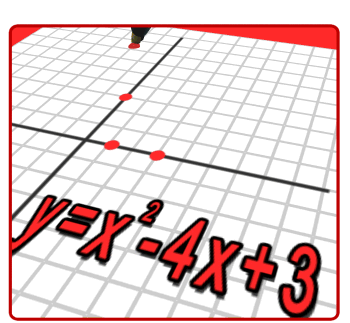•Welcome to Senior Math!

Objectives

The students will demonstrate how to:

• calculate weekly gross wages involving regular pay, overtime pay, and piecework earnings
• calculate earnings for straight commission, or base wage plus commission
• determine the difference between gross pay and net pay calculate weekly, monthly, and yearly net pay
• define and explain the purpose of a budget determine and calculate monthly fixed expenditures
• investigate the guidelines in developing a budget
• plan a budget based on percentages allotted to various categories as suggested by financial institutions
• calculate the portion of total income spent on each category using percents
• draw graphs (including circle graphs) of budget figures using appropriate software
• calculate the actual amount of money to be spent on each category using predetermined percentages
• adjust a budget to changes in expenses.